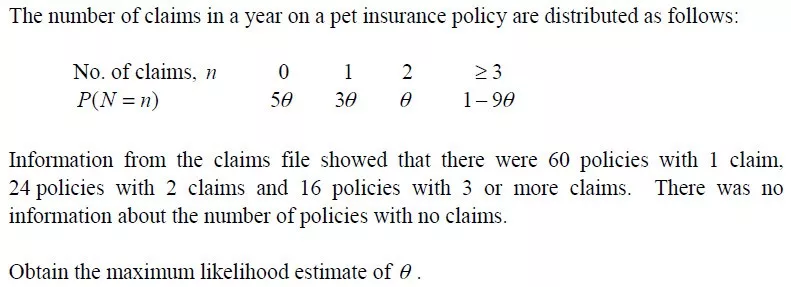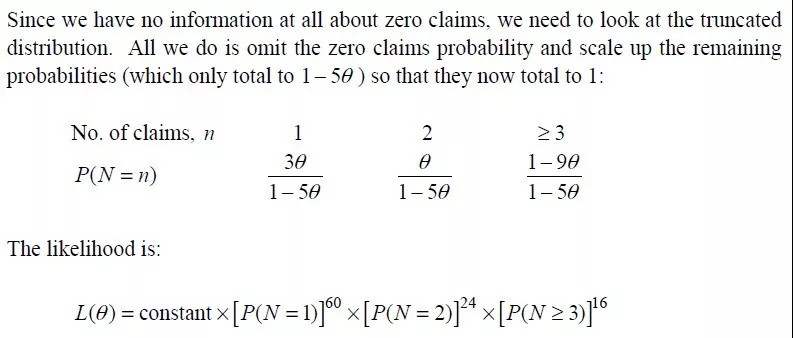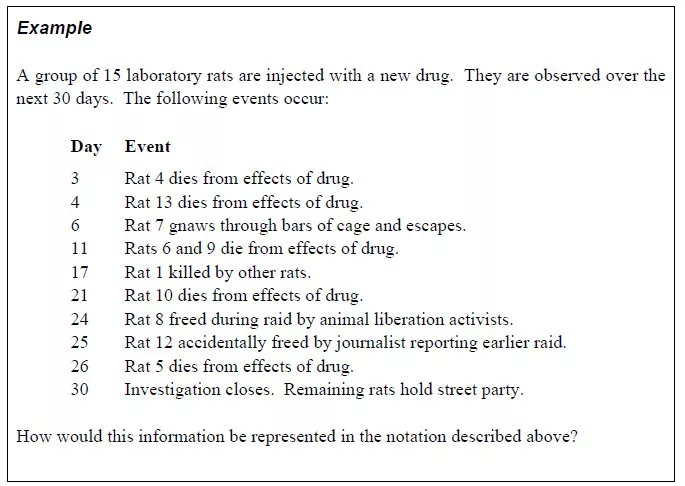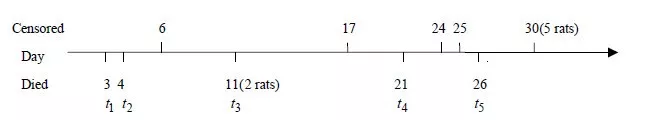CT4-08 Estimating the lifetime distribution function中，对删失数据的概念和分类的系统介绍。（属于生存分析的内容）通过小白鼠的例子介绍卡普兰·迈耶生存分析法（乘积极限法）。

## 1

“这么热要不给它买杯琪琪家的冰豆浆吧。”我这样想着，就走出了实验室，并给它打开了笼子，“这样它应该也会凉快些。”

## 2

〔9〕和〔10〕也是关于小白鼠的后续，算是讲了一个完整的故事。如果想先看完故事，可以直接滑到最后。

## 3

### 删失（Censoring）

Censored data arises when an individual’s life length is known to occur only in a certain period of time.

### 左删失（Left censoring）

• 当估计某个确定的年龄的生存函数，但你不知道准确的出生日期时；
• 当估计某个确定的保险期间的生存函数，但你不知道准确的保单起始日期时；
• 当估计某种疾病的持续期间的生存函数，但你不知道准确的开始生病的时间时。

### 右删失（Right censoring）

• 寿险的投保人退保时；
• 养老金计划的被保险人在退休领取养老金时为健康状态（active）；
• 生死两全保险的保单期满。

### 区间删失（Interval censoring）

• 当你只知道退保的会计年度（calendar year ）时。
• 当估计某个确定的年龄的生存函数，但你只知道死亡发生时的年龄是“x nearest birthday”时。也就是说你只知道死亡发生在【x-0.5，x+0.5】的年龄区间（Rate interval）内；
• 当你知道死亡和出生的会计年度时。因为此时我们有的数据只是“age next birthday at the 1 January prior to death”，我们只知道死亡发生在这个时间区间内。

### 随机删失（Random censoring）

• 寿险的投保人退保时；
• 人群发生迁移；
• 企业年金计划的成员跳槽到另一家企业。

### Informative and non-informative censoring

non-informative censoring的例子：

• 调查期间的结束（因为这对于所有的个体的影响是相同的）。

Informative censoring的分析则比较复杂。（对，你可以把这句话大致理解为Informative censoring只要掌握概念就好）

Informative censoring的例子有：

• 寿险的退保。因为退保的人可能会比没有退保的人健康状态略好，死亡率低一些；
• 养老金计划的被保险人在退休领取养老金时为生病状态（Ill-health）。因为他们的健康状态一般会比留在养老金计划中的人差，死亡率更高。

## 4

CT4对截尾并没有详细讲解，但这里还是补充一下。

### 截尾/截断（Truncation）

Truncation of survival data occurs when only those individuals whose event time lies within a certain observational window (YL,YR) are observed. An individual whose event time is not in this interval is not observed and no information on this subject is available to the investigator.

## 6

### 极大似然估计法的基本步骤

$L(\theta)=\prod_{i=1}^{n} f\left(x_{i} ; \theta\right)$

$\frac{\partial}{\partial \theta} \ln L(\theta)=0$

$\frac{\partial^{2}}{\partial \theta^{2}} \ln L(\theta)<0 \Rightarrow \max$

### Censored data的似然函数

$L(\theta)=\left[\prod_{i=1}^{n} f\left(x_{i} ; \theta\right)\right] \times[\mathrm{P}(\mathrm{X}>y)]^{\mathrm{m}}$

### Truncated data的似然函数## 7

$\hat{\lambda}_{j}=\frac{d_{j}}{n_{j}}(1 \leq j \leq k)$

$\lambda_{j}=P\left[T=t_{j} | T \geq t_{j}\right]$

$F(t)=1-\prod_{t_1\leq t}\left(1-\lambda_{j}\right)$

$\hat{F}(t)=1-\prod_{t_{1} \leq t}\left(1-\hat{\lambda}_{j}\right)=1-\prod_{t_{1} \leq t} \frac{n_{j}-d_{j}}{n_{j}}$

## 8## 9“你我相逢在黑夜的海上